# Pandas Indexing: A Beginner's Guide to Data SelectionUpdated:

Published:

pandas is an open-source library for Python built to simplify manipulating and updating relational or labeled data. It imports data from various file formats and is optimized for fast querying and processing.Once you have data inside pandas, you will most likely need to access segments of the data for different analyses and validations. However, when you have a data set that's hundreds of rows long, simply looking at the raw data trying to find the records you need won't cut it. This is where pandas' indexing methods step in to allow you to pull the exact amount of data you need.

This post will cover indexing methods in pandas two main data structures: Series and DataFrames. Then you will learn more advanced indexing capabilities with partial string indexing and logical indexing for additional ways to find the right information for your analysis.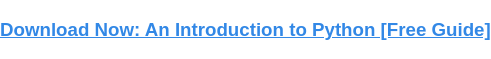## Pandas Indexing: Series

A Series is a one-dimensional array of data. It can hold data of any type: string, integer, float, dictionaries, lists, booleans, and more. The easiest way to conceptualize a Series is a single column in a table, which is why it's considered one-dimensional.

Here you can see the result of printing a Series to the terminal. This Series was created from a list, so the underlying data type (dtype) is an object because lists are considered objects in Python.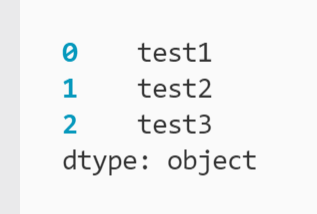Series is the simpler data structure, so we'll start here to introduce the basics of indexing in pandas before moving on to DataFrames. First, let's look at default index behavior.

### Default Index in Pandas Series

If you look at the example pandas Series in the last section, you'll see numbers on the left-hand side starting at zero. These are the index values of the Series. The index is a reference point for each record. In other words, we use an index to tell Python which data value we want to retrieve.

``` ```
print(myseries)
``````

Here, the command says to retrieve the data point in myseries at position 0. The index is denoted with the square brackets ([ ]), which is known as the indexing operator. Because pandas Series and DataFrames are zero-indexed, you are selecting the first value when you reference the index value 0. You can see the result of this operation using the print() function.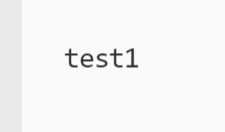### Custom Index in Pandas Series

As you have seen, indexes for Series are integers by default (this is also true for DataFrames in the later section). But what if you want to search by the data values themselves? This is where custom indexes come in handy.

To create a custom index, you can define the index when first creating the Series:

``` ```
myseries = pd.Series(myseriesdata, index = myseriesdata)
``````

This creates a Series from the list myseriesdata and sets the index equal to the values of myseriesdata. The result is printed below.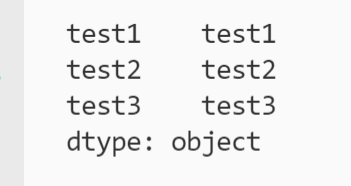Now you can search with both integers and strings. Strings are referred to as labels when referencing indexes.

``` ```
myseries["test2"]
myseries
``````

If you run both these commands, the output will be the same: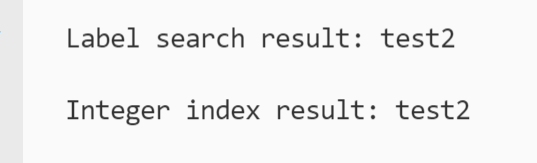Note that "Label search result" and "Integer search result" are strings added to the print statements to distinguish the results. Also note that labels are case sensitive, so if you tried to find the record at the "Test2" position, you would receive a KeyError.

Selecting data from Series with labels might seem redundant. After all, if you already know what the value is to specify it, what's the point of then searching for it? Labels may not be as important with Series, but they will become immensely useful in DataFrames, so keep this concept in mind.

Custom index labels also open up a new function to simplify your indexing: .loc.

### .loc Indexing in Pandas Series

The .loc (short for location) function was created to select data by the index label. You can see an example implementation below.

``` ```
myseries.loc["test2"]
``````

This produces the same output as the earlier example of label indexing without the .loc function.

You might wonder why this function is necessary if you can search by the label with the base indexing operator. .loc was implemented to prevent confusion by indexing only with labels. Now let's examine this function's counterpart: .iloc.

### .iloc Indexing in Pandas Series

.iloc (short for integer location), selects data only with integer indexes.

``` ```
myseries.iloc
``````

Once again, the result is the same as the previous examples of default indexing.

Together, .loc and .iloc explicitly declare which method is being used to index the Series. Explicit code is better because it is easier for new coders to read and understand what is happening in a program. It can even be helpful for the code authors when debugging.

There is a third indexing option available, .ix, that accepts both integers and labels. However, this function has been deprecated since pandas version 0.20.0, so just be aware that you may encounter this method in older code.

Let's move on from Series and look at the second data structure, DataFrames, where the power of indexing shines through.

## Pandas Indexing: DataFrames

DataFrames are two-dimensional structures that display data in rows and columns. A DataFrame is the pandas equivalent of a table, as you can see below.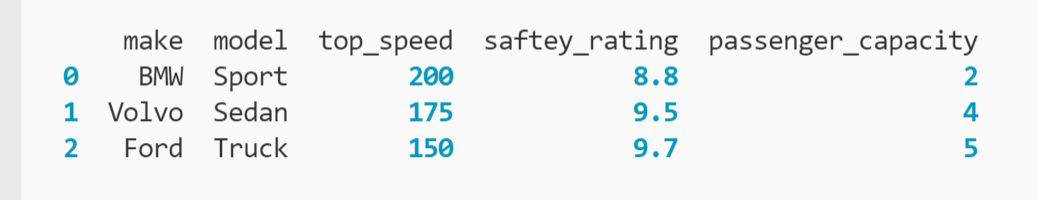Each column in a DataFrame can be considered a Series (i.e. if you extract one column, it becomes a Series). Now you have both row and column indexes to select data with.

### Default Index in Pandas DataFrames

Before diving into custom indexes, let's take a quick look at the default index with DataFrames. Your options are limited to indexing columns as opposed to records with Series.

To index a single column, use the column name with the indexing operator:

``` ```
mydataframe["model"]
``````

To index multiple columns, place a list of column names inside the initial brackets:

``` ```
mydataframe[["model", "make"]]
``````

If you print the result, note that the column order follows your list's order, regardless of the column order in the original DataFrame.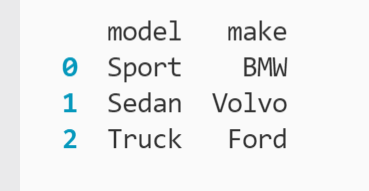Now, let's revisit custom indexes.

### Custom Index in Pandas DataFrames

Like with Series, you can assign a custom index when first creating the DataFrame:

``` ```
mydataframe = pd.DataFrame(mydataset, index = mydataset["make"])
``````

In addition, you can also assign a custom index after the DataFrame is generated using the .set_index method:

``` ```
mydataframe.set_index("make", inplace = True)
``````

This function assigns the values of an existing column to the index in the DataFrame, which we specify with the column name (make). By default, set_index creates a new copy of the DataFrame; setting the inplace parameter equal to True reverses this behavior so that mydataframe is modified "in place." You can see the result below.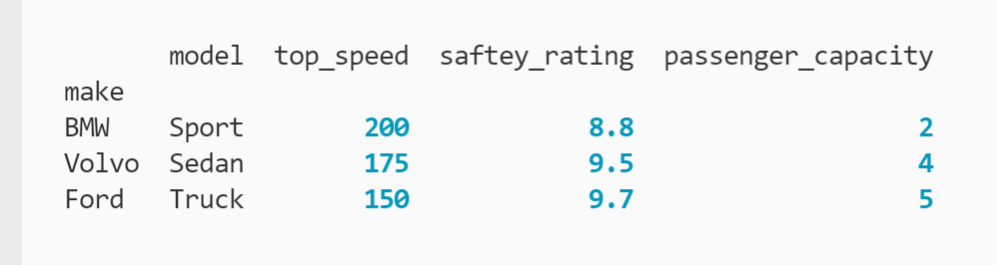However, now any records indexed will not include the make values, since it is no longer a column. If you want to make a column an index while still maintaining the original column, set the drop parameter's argument to False:

``` ```
mydataframe.set_index("make", drop = False, inplace = True)
``````

Printing the modified DataFrame confirms that the column has not been dropped to create the index.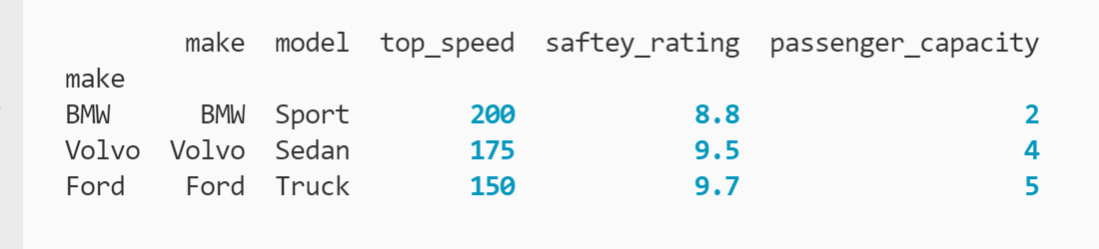### .loc Indexing in Pandas DataFrames

Using the .loc function, you can select a single row by its label with ease:

``` ```
mydataframe.loc["BMW"]
``````

You can see the output below.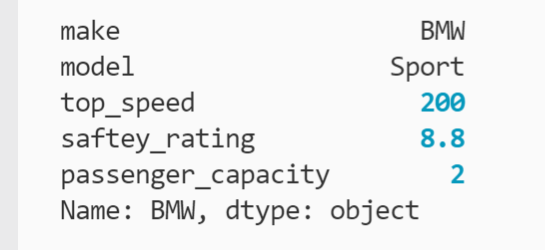You can select multiple rows with a list the same way you select multiple columns with the indexing operator:

``` ```
mydataframe.loc[["BMW", "Ford"]]
``````

In DataFrames, you have a whole new dimension to segment data by: the column. You provide a second list as a parameter if you only want to include certain columns:

``` ```
mydataframe.loc[["BMW", "Ford"], ["model", "safety_rating"]]
``````

Here, you have selected the rows with indexes BMW and Ford and specified the columns model and top_speed. The result provides this exact segment:Finally, you can pull all rows and only some columns. To do this, use the colon (:) symbol for the row argument:

``` ```
mydataframe.loc[:, ["model", "safety_rating"]]
``````

The result gives you all rows and the named columns: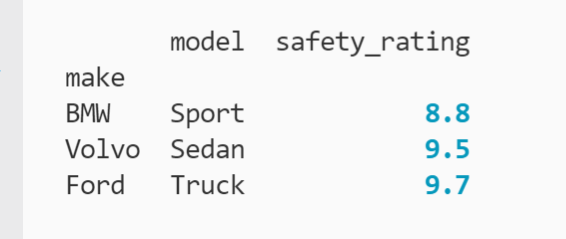### .iloc Indexing in Pandas DataFrames

The .iloc function follows the same conventions as .loc for DataFrames, except with integers for the index. You can pull a single row:

``` ```
mydataframe.iloc
``````

You can pull multiple rows with a list:

``` ```
mydataframe.iloc[[0, 2]]
``````

You can select specific rows and columns using lists:

``` ```
mydataframe.iloc[[0, 2], [1, 2, 4]]
``````

Where we'll focus our attention for .iloc is a new segmentation method: slicing. With slices, you can specify a range of rows or columns. If you want to select the first two rows (and all columns), use the colon with the corresponding integer range:

``` ```
mydataframe.iloc[0:2]
``````

The result is below.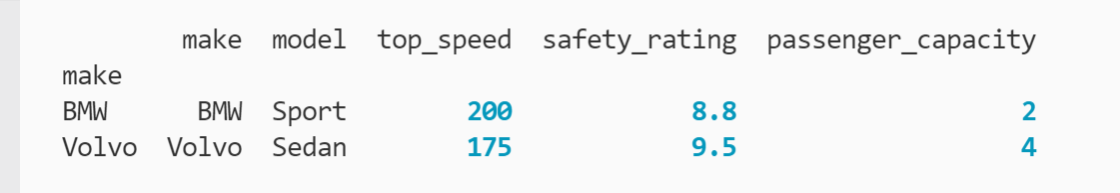You may notice that there is no third record even though our range terminates at index 2. This is because our range is exclusive, meaning that our slice includes everything up to index 2. You will often see this term (and its counterpart inclusive) used when discussing number ranges in programming.

You can also specify a range of columns as a slice:

``` ```
mydataframe.iloc[0:2, 2:4]
``````

In this example, the ranges specify the first two rows and the third and fourth columns. The output is below.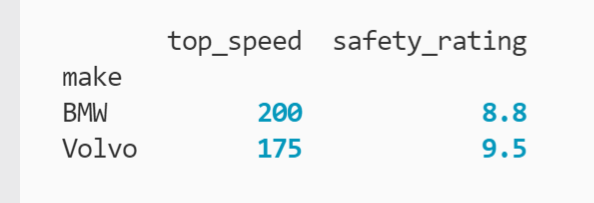Note that slices can also be used with .loc indexing, but it's more common to use ranges with .iloc since you would need to know the start and ending labels of each range, which would require you to know your DataFrame's row and column labels. Plus, this approach is more prone to errors with typos.

Now that you understand the main indexing methods in pandas, let's take a look at two advanced use cases, starting with partial string indexing.

## Partial String Indexing in Pandas

Partial string indexing allows us to better understand the similarities between different records based on their index value. For example, below is a DataFrame quantifying the count and audience base of different cinema offerings in a media library.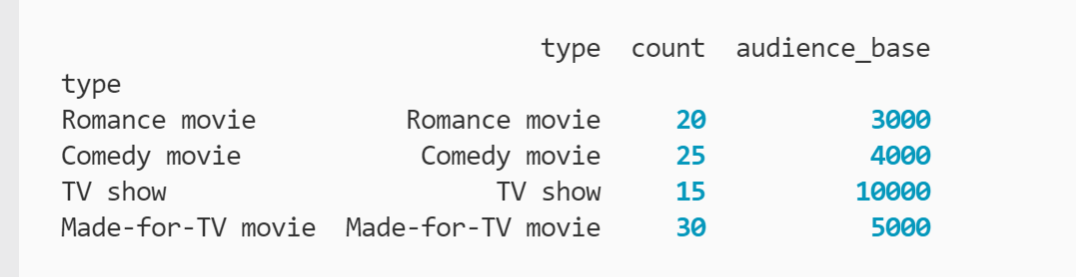You want to find records that have a type related to TV, so you segment the data by looking for indexes that contain the phrase "TV". Because the code only looks at part of an index's label (AKA string), this approach is known as partial string indexing.

``` ```
mydataframe2.loc[mydataframe2.index.str.contains("TV")]
``````

Here you are providing the .loc[] function a list of boolean values based on which index values include "TV" by using the .contains() method of the string (.str) methods of the DataFrame's .index property. You can see a printout of the list below:The result of providing this list to .loc is below.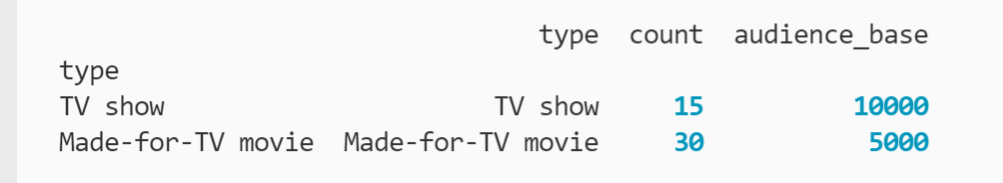Just like with regular label indexing, the argument provided to .contains() is case sensitive. This means partial string indexing for "tv" would return no results.

In the next section, let's examine how to apply booleans and logical operators to pandas indexing.

## Logical Indexing in Pandas

If logical indexing sounds intimidating, guess what? You've already done it if you followed the example in the partial string indexing section. In that use case, we used the contains method to create a list of boolean values by determining which indexes included the string provided. You can see the result of the contains operation below.

``` ```
mydataframe2.loc[[False, False, True, True]]
``````

By providing a list of true or false values, .loc[] will return the rows that correspond to the True booleans — the third and fourth rows in this case.

Filtering based on a list of boolean values is known as boolean masking.

Let's take a look at a couple more use cases.

### 1. Custom Boolean Index

Beyond masking, you can also define a custom index with boolean values. This can either come from an existing column of boolean values after creating the DataFrame or from a list of booleans while creating the DataFrame. For this example, the index is defined during creation:

``` ```
pd.DataFrame(mydataset2, index = [True, False, True, False])
``````

The result of this command is below.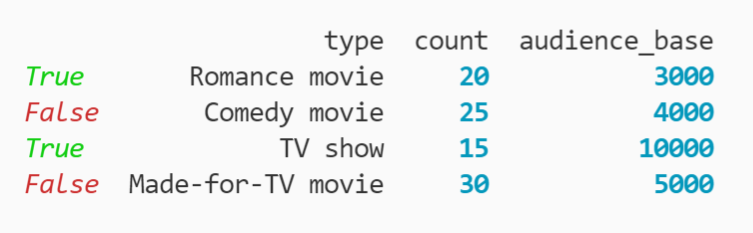Now, you can use .loc[] to filter out rows that have a true or false index:

``` ```
mydataframe2.loc[True]
# OR
mydataframe2.loc[False]
``````

Note that providing a boolean value to .iloc will result in a TypeError.

Next, let's look at how you can index with relational operators.

### 2. Relational Operator Indexing

You can filter your data based on comparisons with the relational operators available in Python. For this example, you can filter out media types whose audience base is less than 5,000 viewers.

``` ```
mydataframe2['audience_base'] >= 5000
``````

The relational operator (>=) is applied to the column audience_base to calculate whether the integers stored in the column are greater than or equal to 5,000. You can see the result of this operation below.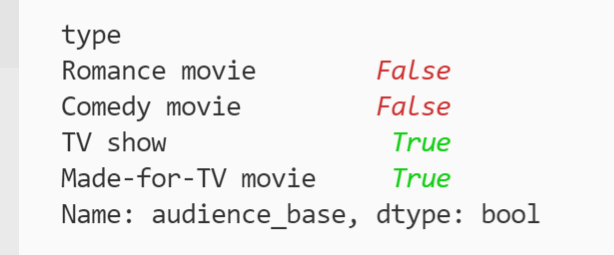The original values of the audience_base column are now masked with boolean values indicating whether they met the 5,000-base audience condition.

## Find the right records every time with Pandas indexing.

Indexing in pandas is an effective and powerful way to segment your data for analysis and export. You can use indexing across Series and DataFrames with default and custom index options. The .loc and .iloc methods offer even greater capabilities to segment, slice, and specify your data selections. Partial string and logical indexing provide even greater flexibility as you dive deeper into your data exploration.Topics: What Is Python?

## Related Articles

We're committed to your privacy. HubSpot uses the information you provide to us to contact you about our relevant content, products, and services. You may unsubscribe from these communications at any time. For more information, check out our Privacy Policy.

A guide for marketers, developers, and data analysts.